# STRAIGHT LINES, INTERMEDIATE FIRST YEAR 1B CHAPTER 3 PROBLEMS WITH SOLUTIONS

Mathematics intermediate 1a and 1b solutions  for some problems are available here. These are very simple to understand.

Inter first year 1a : Functions, mathematical inductions, addition of vectors, product of vectors and trigonometry.

Inter first year 1b : Locus, transformation of axes, the straight line, pair of straight lines, three dimensional coordinates, direction cosines & ratios and applications of derivatives.

The Straight line

You can see the solutions of junior inter 1b

1Locus

The straight line sa

Straight lines la

12. Rate measure

You can also see the solutions of junior inter 1A

3. Matrices

You can see the solutions for text book Maths 1A

Functions

Exercise 1(a)

Exercise 1(b)

Exercise 1(c)

Mathematical Induction

Exercise 2(a)

Matrices

Exercise 3(a)

Exercise 3(b)

Exercise 3(c)

Exercise 3(d)

Exercise 3(e)

Exercise 3(f)

Exercise 3(g)

Exercise 3(h)

Exercise 3(i)

Exercise 4(a)

Exercise 4(b)

Product of vectors

Exercise 5(a)

Exercise 5(b)

Exercise 5(c)

Trigonometric Ratios up to Transformations

Exercise 6(a)

Exercise 6(b)

Exercise 6(c)

Exercise 6(d)

Exercise 6(e)

Exercise 6(f)

Trigonometric Equations

Exercise 7(a)

Inverse Trigonometric Equations

Exercise 8(a)

Hyperbolic Functions

Exercise 9(a)

Properties of Triangles

Exercise 10(a)

Exercise 10(b)

Maths 1B solutions for

Locus

Exercise 1(a)

Transformation of Axes

Exercise 2(a)

The Straight line

Three Dimensional Coordinates

Exercise 5(b)

Direction Cosines and Direction Ratios

Exercise 6(a)

Exercise 6(b)

The Plane

Exercise 7(a)

Limits and Continuity

Exercise 8(a)

Exercise 8(b)

Exercise 8(c)

Exercise 8(d)

Exercise 8(e)

Applications of Derivatives

# JUNIOR INTER COORDINATE GEOMETRY STRAIGHT LINES

### Very short answer questions and solutions

Problem 1Problem 2

##Problem 3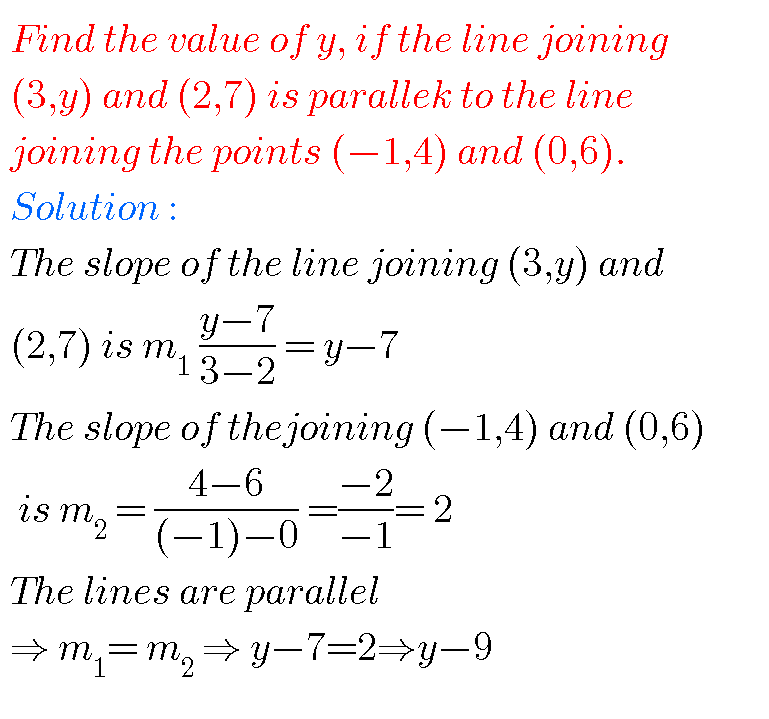Problem 4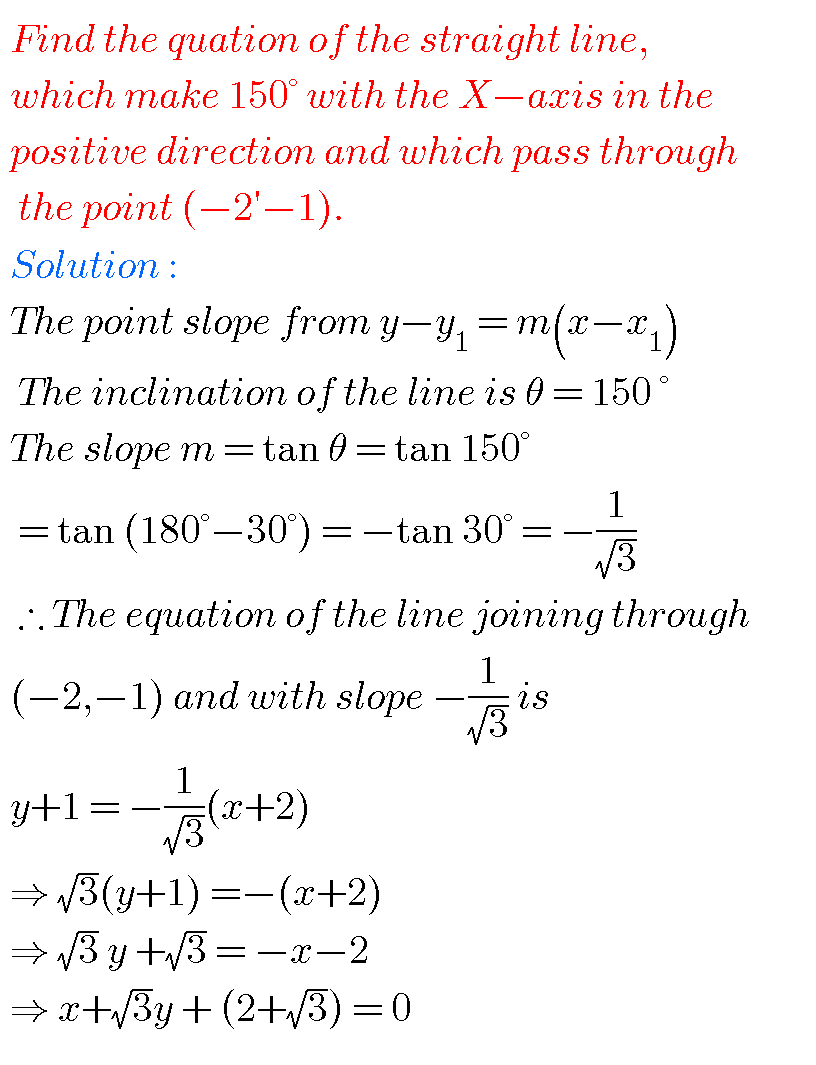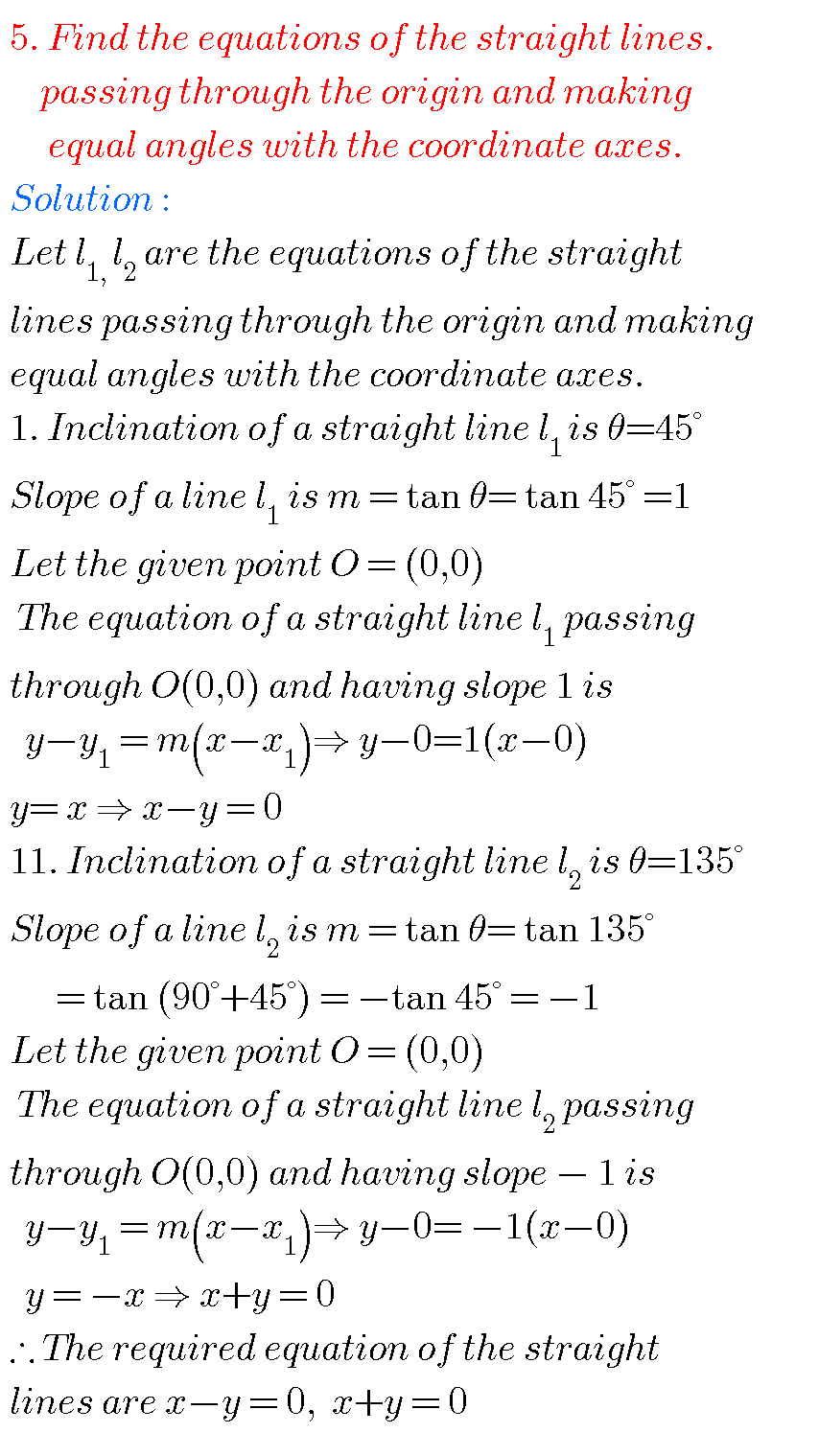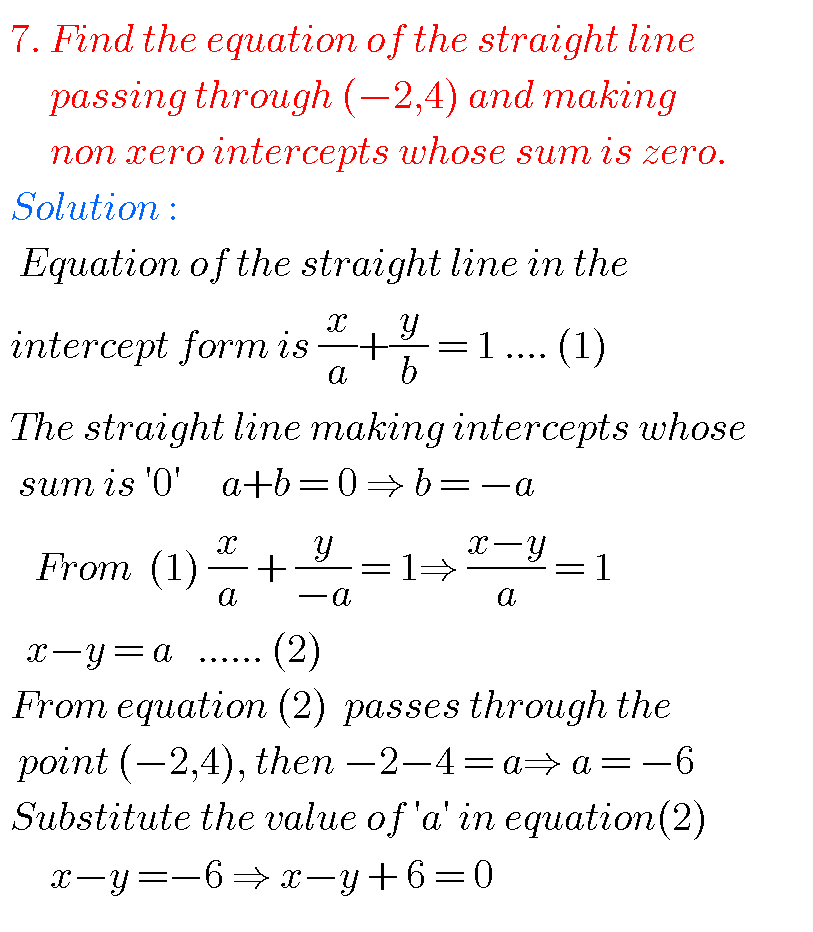##Problem 10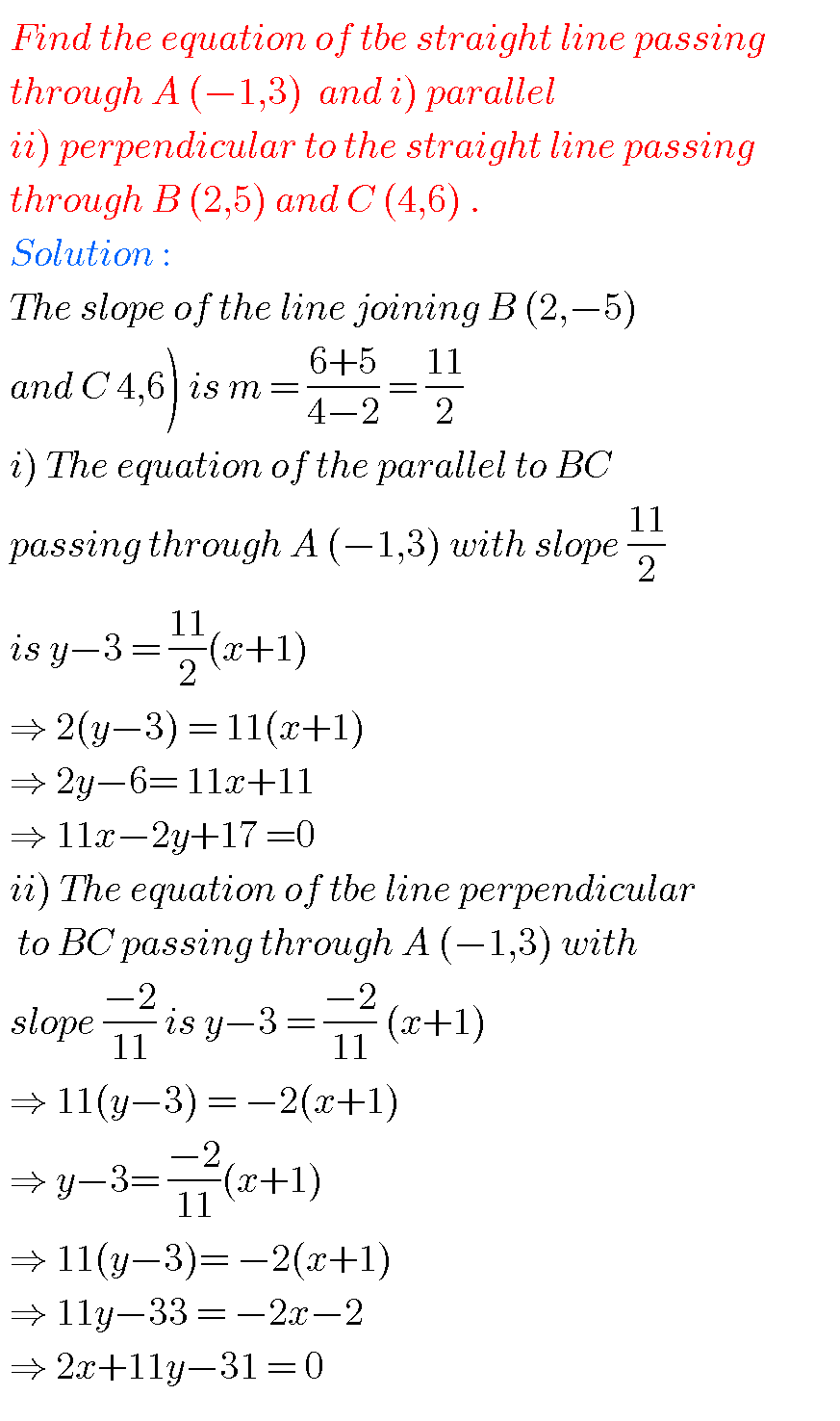##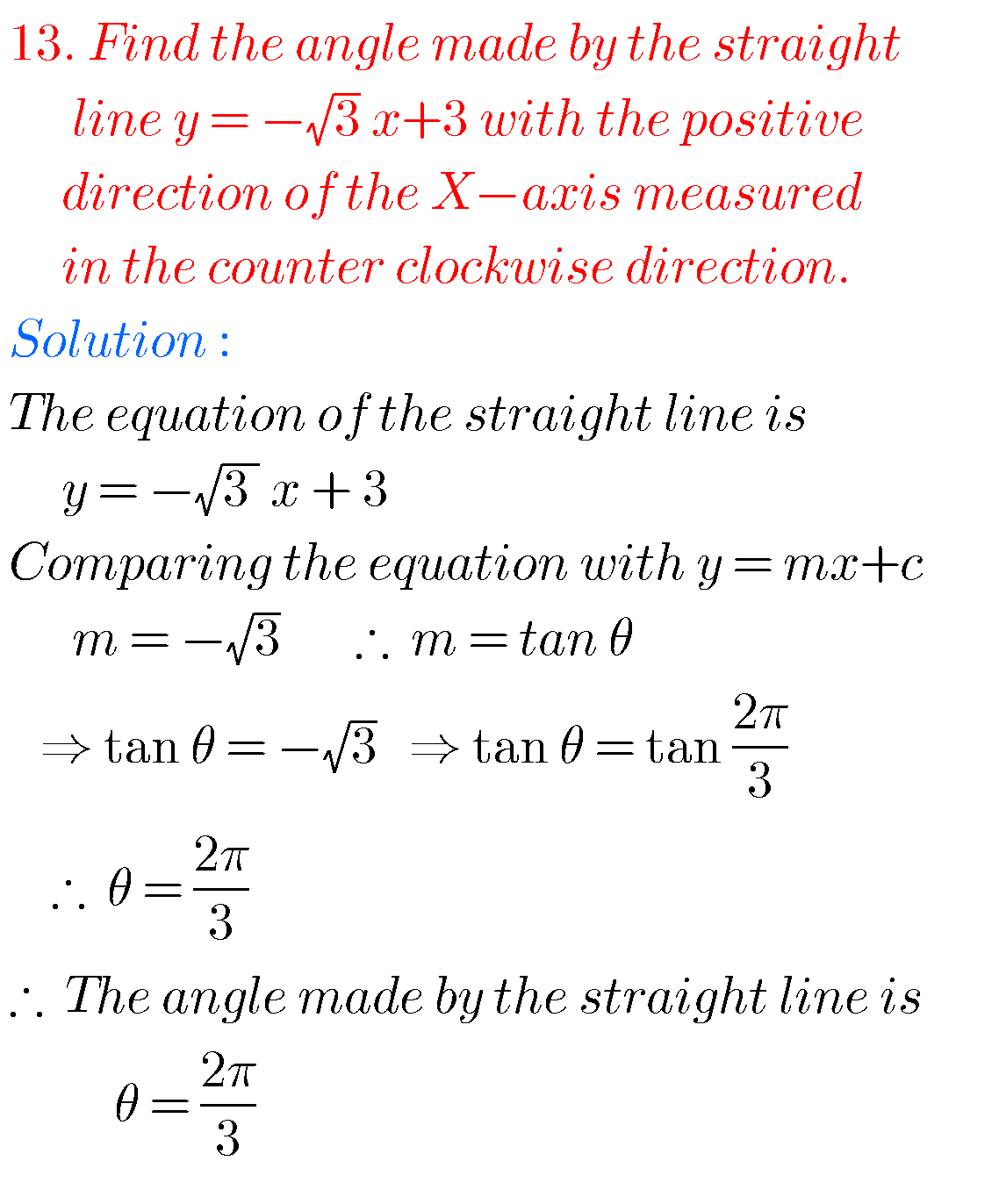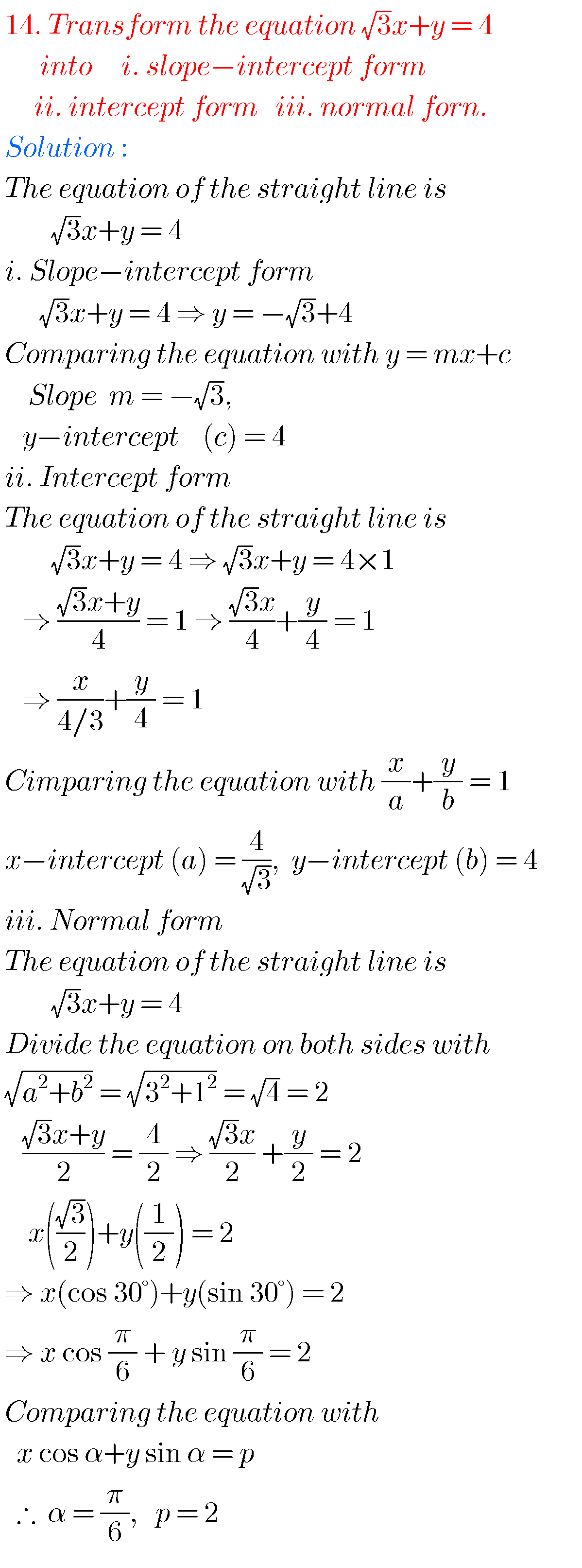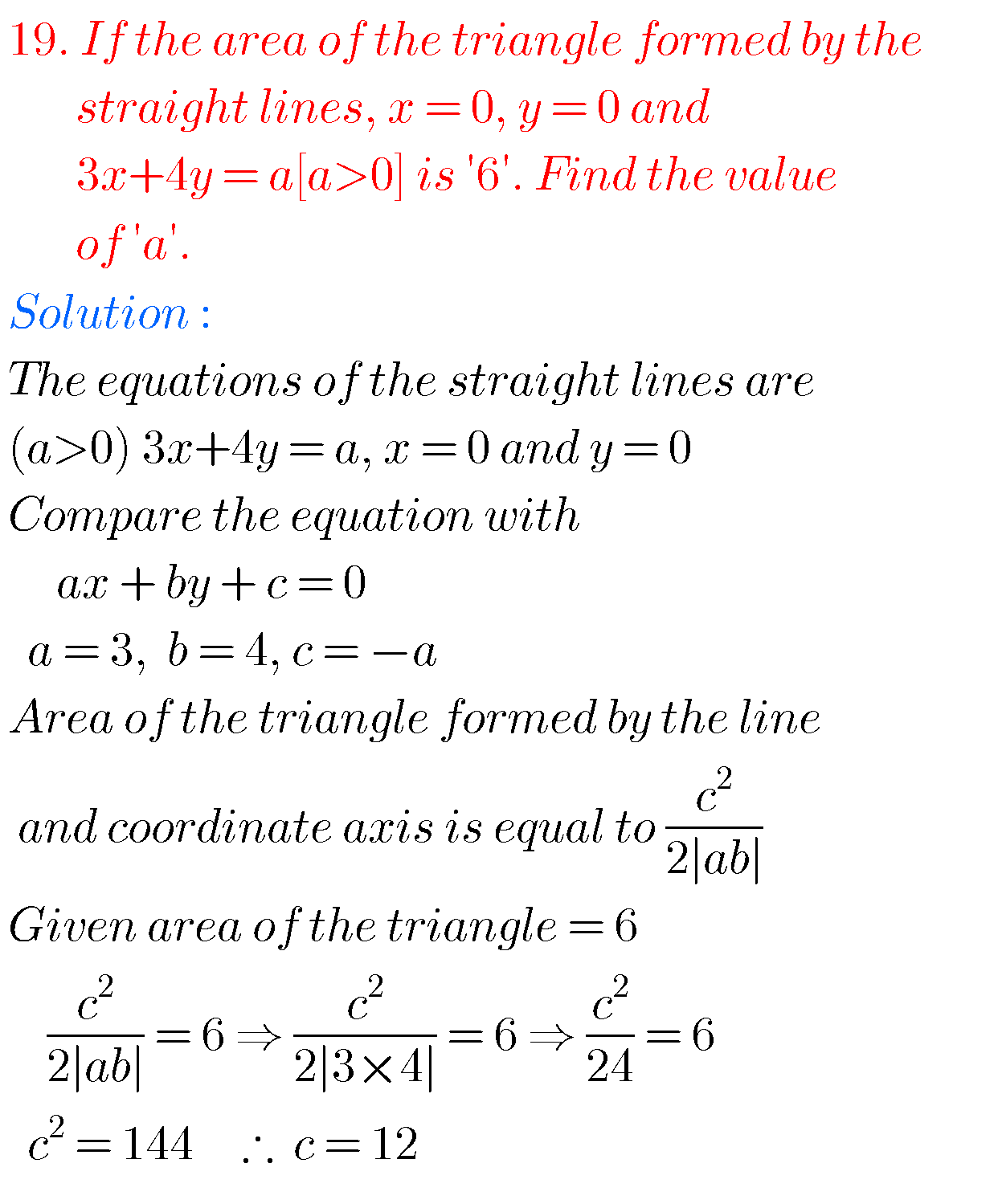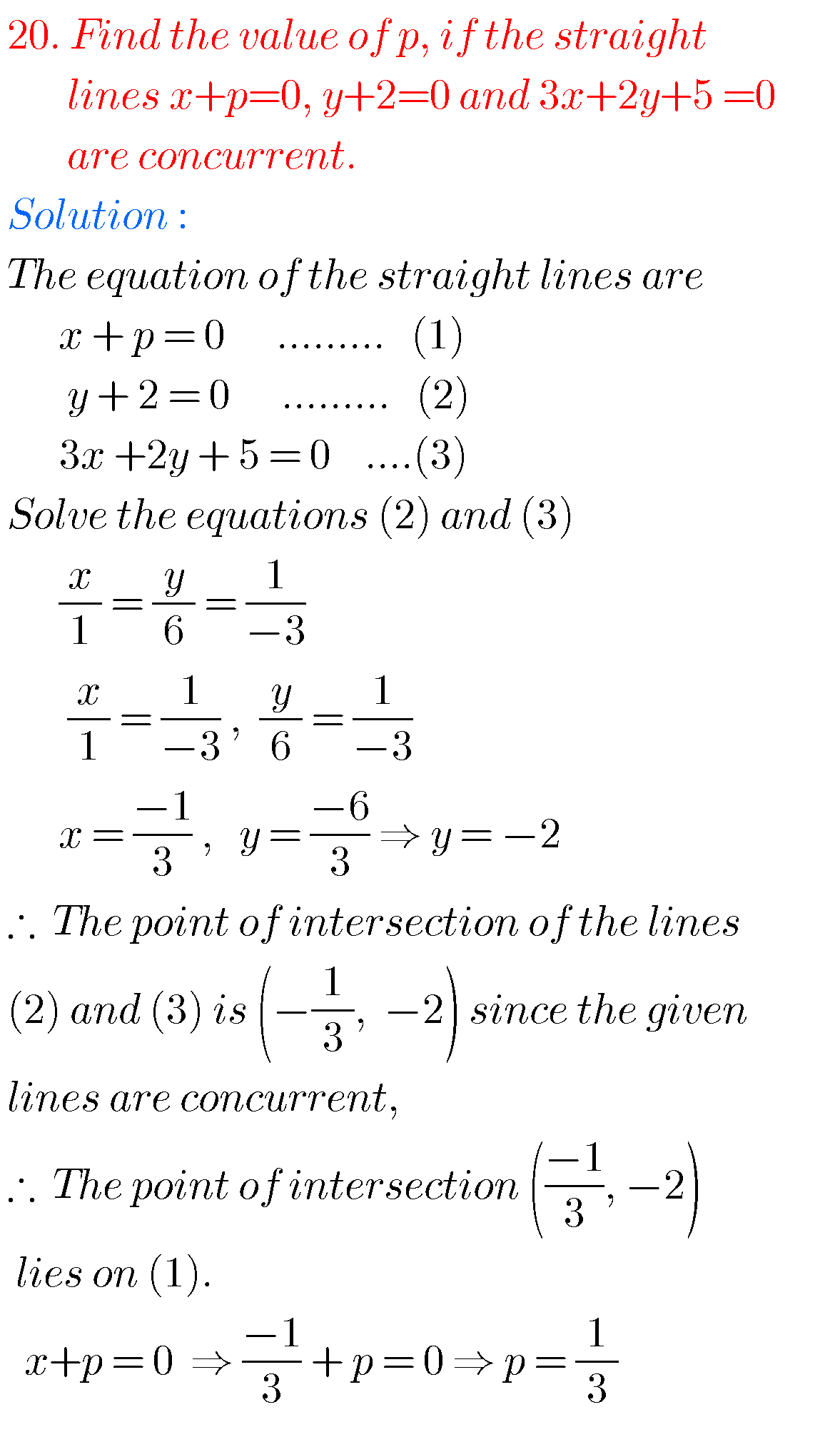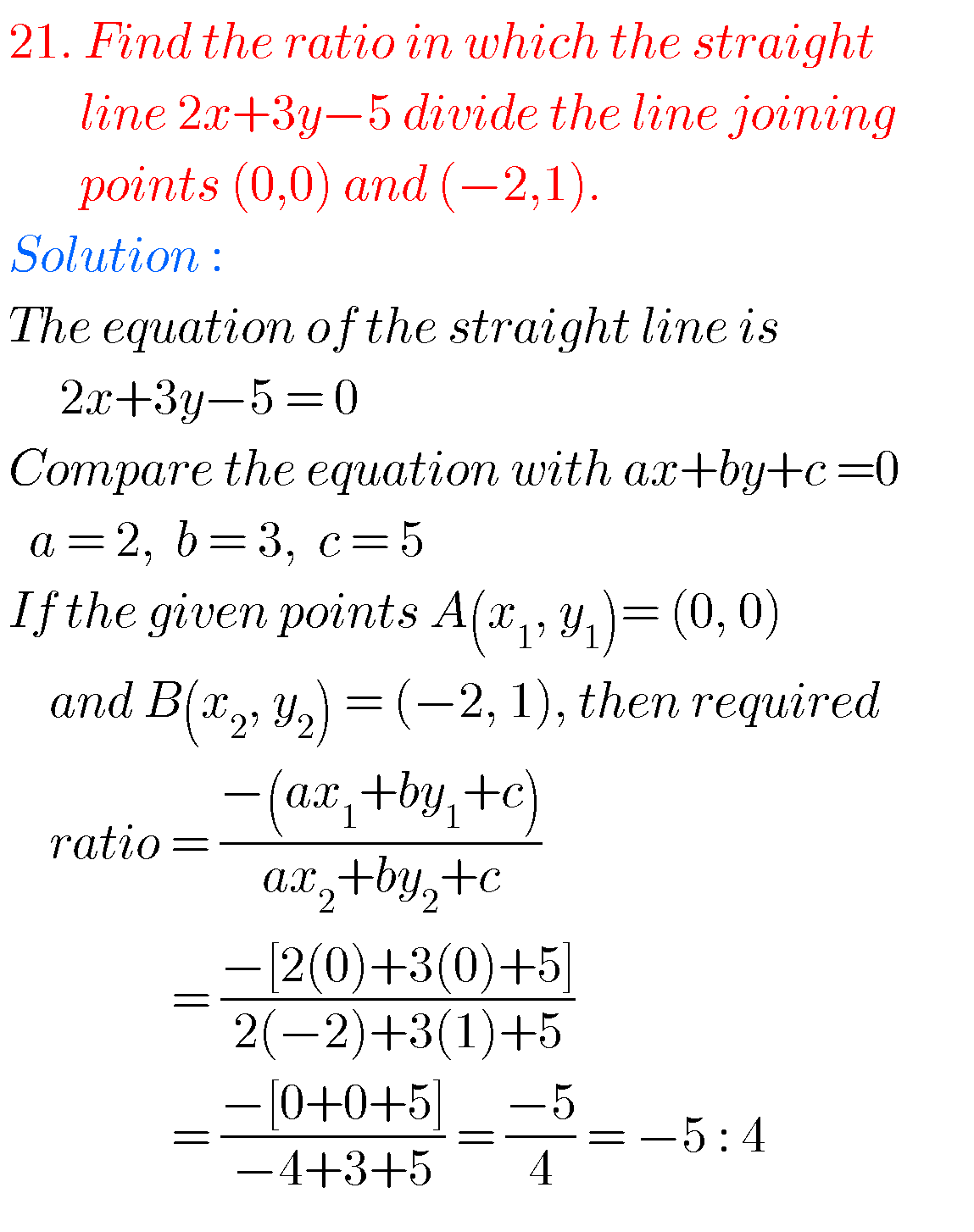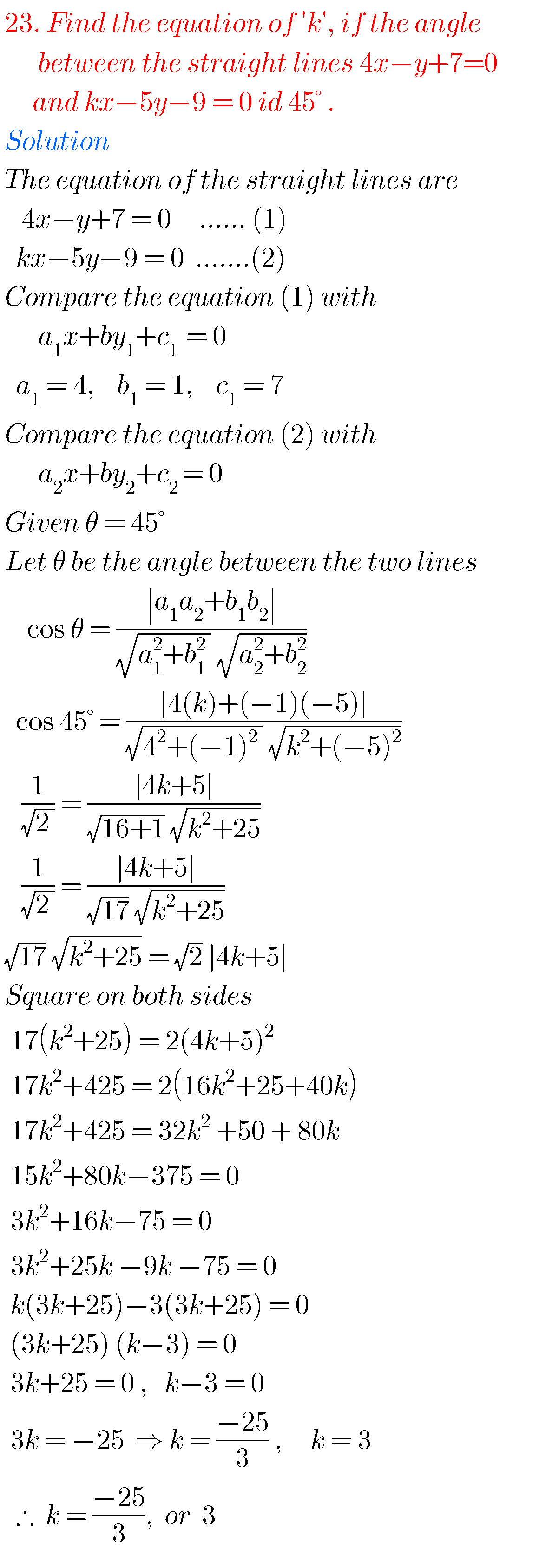Problem 24Problem 25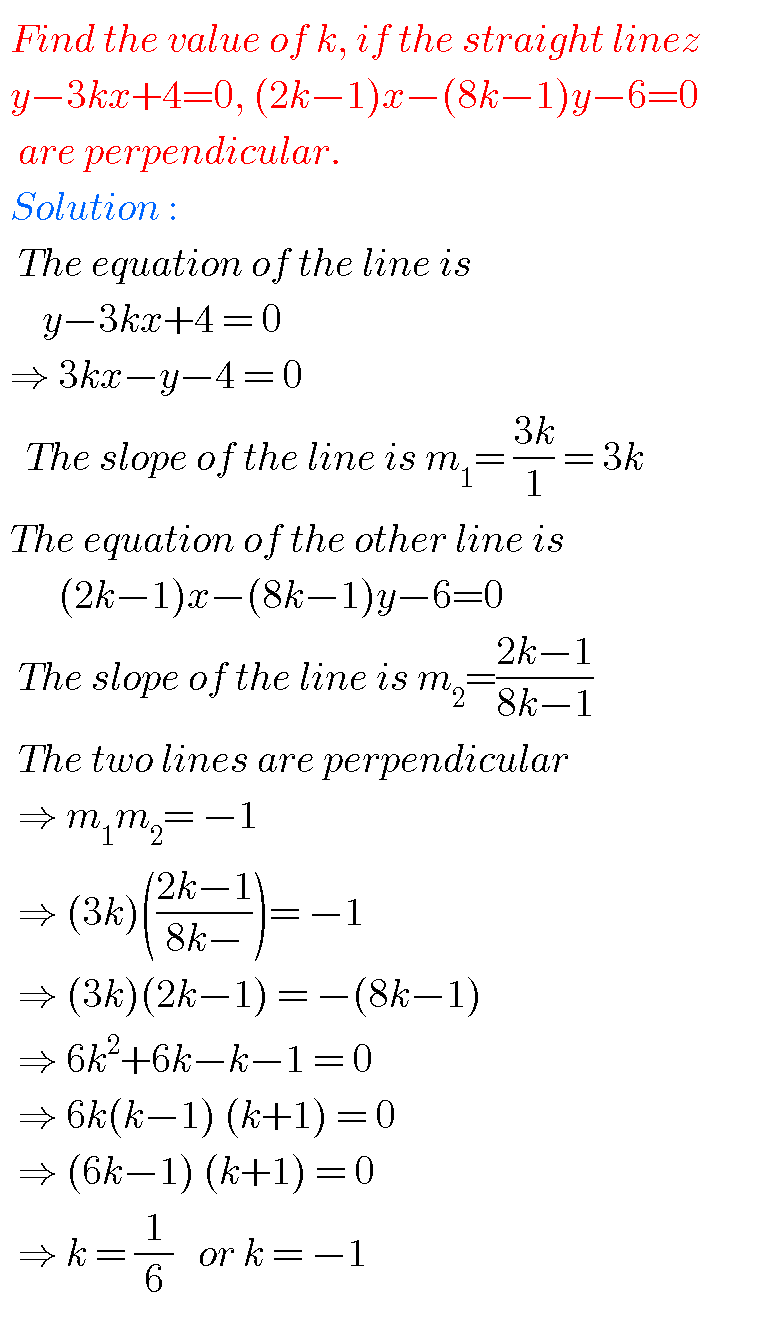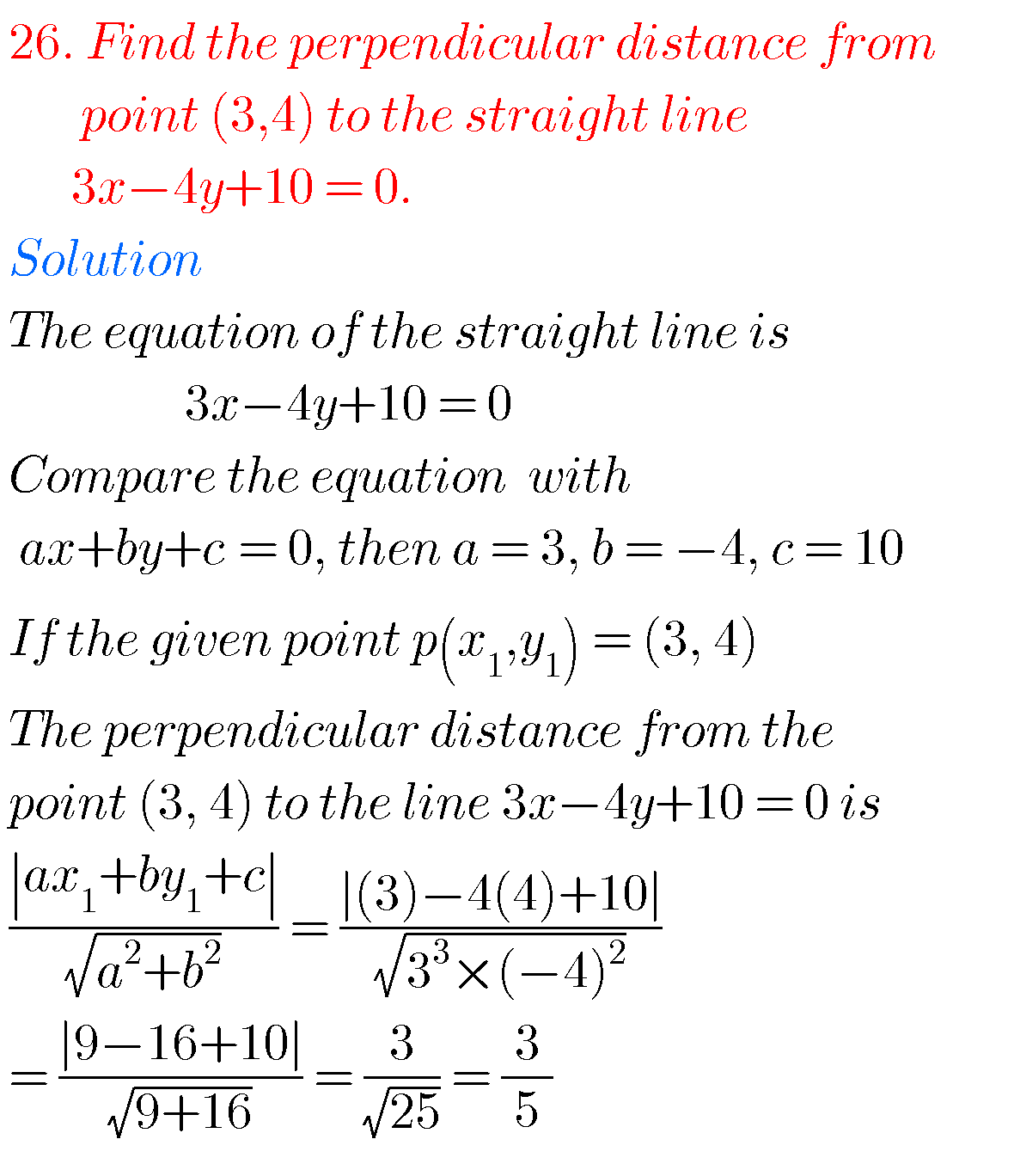## JUNIOR INTER COORDINATE GEOMETRY STRAIGHT LINES

Some more problems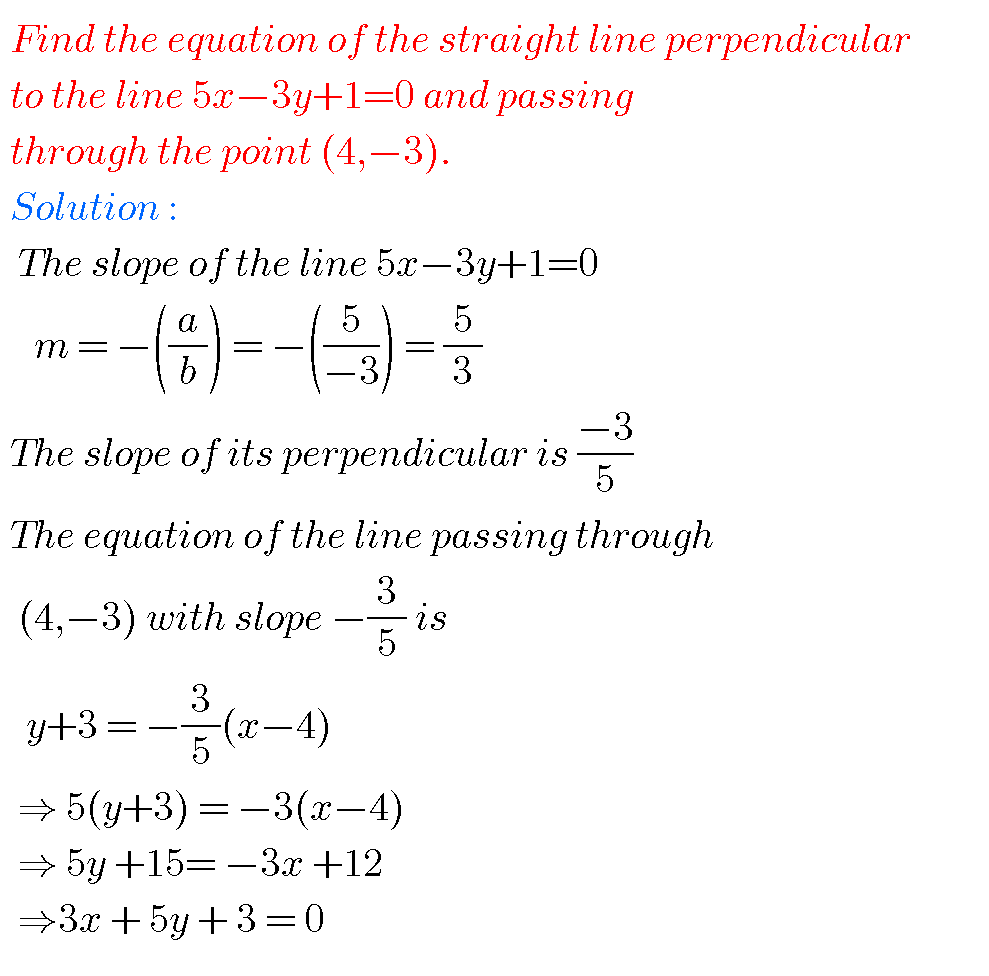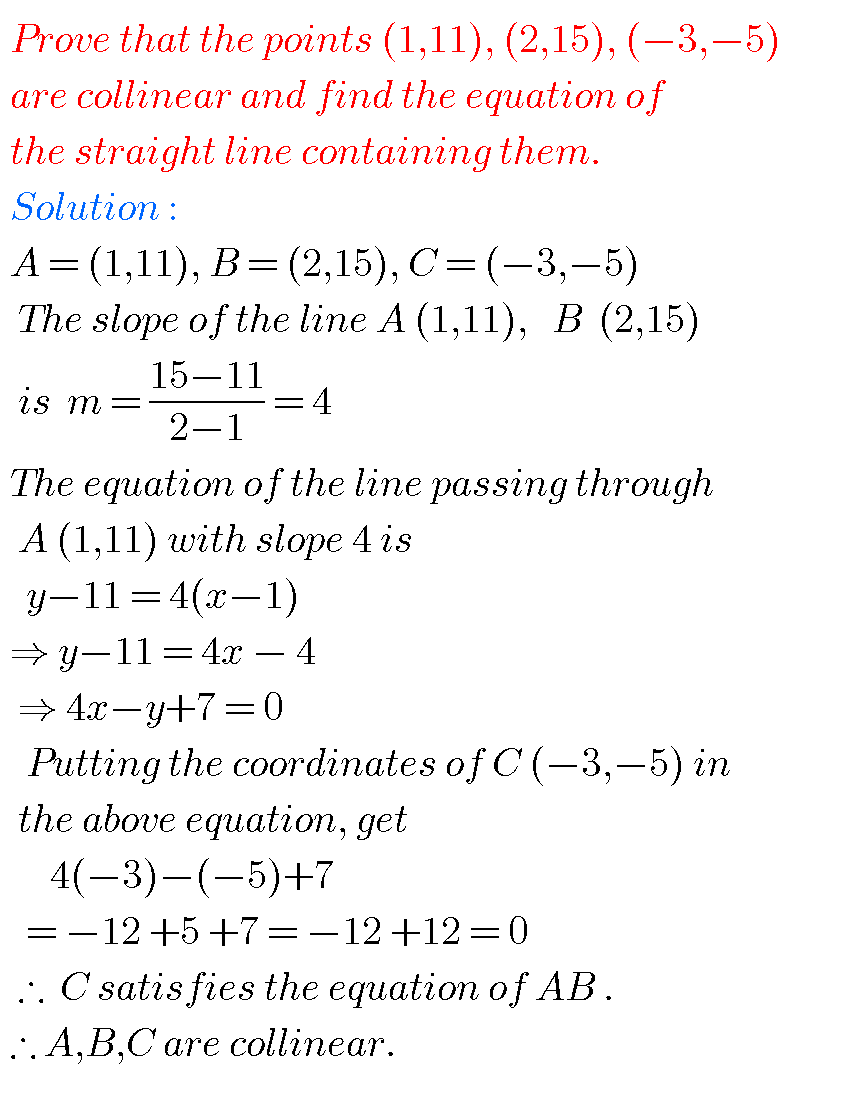Note : Observe the solutions and try them in your own methods.

The straight line short answer questions solutions

Inter maths 1a solutions

Inter maths trigonometry solutions

Maths real numbers solutions class 10

Intermediate maths 2a solutions textbook

You can see solutions for Inter Maths IIB

1. Circle

3. Parabola

4. Ellipse

You can also see solutions for Inter Maths IIA

For examination purpose you can see

Complex numbers

De Moivre’ s Theorem

Ncert Maths class 6 chapter 14 solutions

1.Mamidi rajender reddy April 11, 2019 at 3:32 pm - Reply

Thank u for given this type of information actually I am trying to search book then I got a book like this now the text book is in my fingers

2.Nikhil August 15, 2019 at 3:47 pm - Reply

Are they important sums?

3.Suresh September 12, 2019 at 12:16 pm - Reply

Nicely

4.Himabindhu December 4, 2021 at 6:15 am - Reply

This is too good.Im understanding this very well